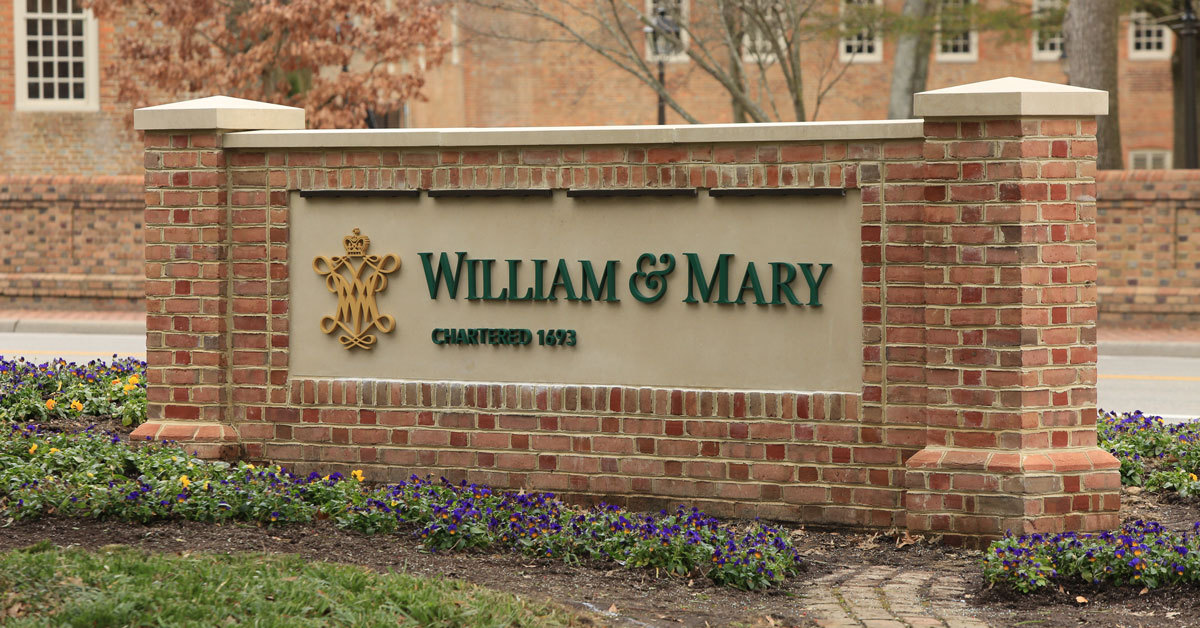Latest about COVID-19 and the Path Forward for fall.# Mathematical Biology

Mathematical Biology aims at modeling natural, biological processes using mathematical techniques and tools. It has both practical and theoretical applications in biological research. Applying mathematics to biology has a long history, but only relatively recently has there been a dramatic increase of interest in the field. Some reasons for this include: the explosion of data-rich information sets, due to the genomics revolution, which are difficult to understand without the use of analytical tools; recent development of mathematical tools such as chaos theory to help understand complex, nonlinear mechanisms in biology; an increase in computing power which enables calculations and simulations to be performed that were not previously possible; and an increasing interest in computer experimentation due to the complications involved in human and animal research.

Students may major or minor in the CAMS - Mathematical biology track.